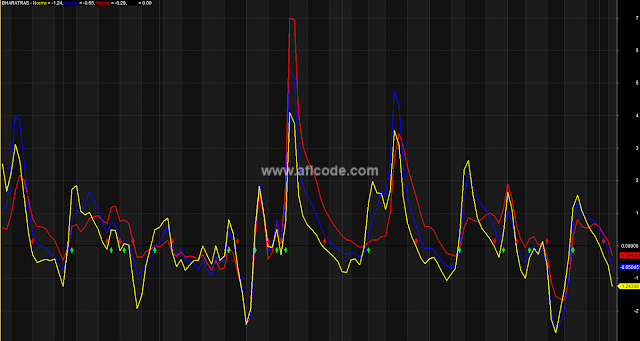### Kernel Entry Exit Trading SystemKernel Entry Exit Trading System

```//www.aflcode.com
function Kernel(Input, Length)
{

Norm = 0;
sigma = (Length+1)/4.0;

for (i = 0; i <= Length; i++)

{
Norm = Norm + exp(-((Length/2 - i)*(Length/2 - i))/(2*sigma*sigma));
}

array = Input;

for (j = Length; j < BarCount; j++)
{
Filtered = 0;

for (k = 0; k <= Length; k++)

{
Filtered = Filtered + exp(-((Length/2 - k)*(Length/2 - k))/(2*sigma*sigma))*Input[j - k];
}

array[j] = Filtered/Norm;

}
return array;
}

function Kernel_HMA(Input, N)
{
f = Kernel(2 * Kernel(Input,round(N/2)) - Kernel(Input,N), round(sqrt(N)));
return f;
}

Length1 = Param("Length1", 8, 2, 50, 1);
Length2 = Param("Length2", 13, 2, 50, 1);
Length3 = Param("Length3", 21, 2, 50, 1);

Smoothx = Kernel(C, Length1);
Smoothy = Kernel(C, Length2);
Smoothz = Kernel(C, Length3);

x = C - Smoothx;
y = C - Smoothy;
z = C - Smoothz;

Normx = Kernel_HMA(x/sqrt(Kernel(x^2,Length1)),2);
Normy = Kernel_HMA(y/sqrt(Kernel(y^2,Length2)),2);
Normz = Kernel_HMA(z/sqrt(Kernel(z^2,Length3)),2);

Buy = ( Cross(Normx,0) OR Cross(Normy,0) OR Cross(Normz,0)) AND (Normx > Ref(Normx,-1) AND Normy > Ref(Normy,-1) AND Normz > Ref(Normz,-1));
Sell = ( Cross(0,Normx) OR Cross(0,Normy) OR Cross(0,Normz) ) AND (Normx < Ref(Normx,-1) AND Normy < Ref(Normy,-1) AND Normz < Ref(Normz,-1));
Short = Sell;